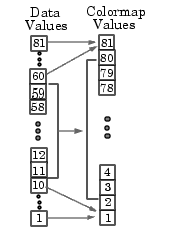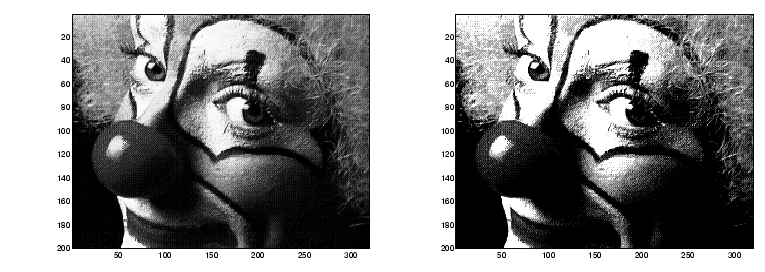MATLAB Function Referenceimagesc

Scale data and display an image object

Syntax

• ```imagesc(C)
imagesc(x,y,C)
imagesc(...,clims)
h = imagesc(...)
```

Description

The `imagesc` function scales image data to the full range of the current colormap and displays the image. (See Examples for an illustration.)

```imagesc(C) ``` displays `C` as an image. Each element of `C` corresponds to a rectangular area in the image. The values of the elements of `C` are indices into the current colormap that determine the color of each patch.

```imagesc(x,y,C) ``` displays `C` as an image and specifies the bounds of the x- and y-axis with vectors `x` and `y`.

```imagesc(...,clims) ``` normalizes the values in `C` to the range specified by `clims` and displays `C` as an image. `clims` is a two-element vector that limits the range of data values in `C`. These values map to the full range of values in the current colormap.

```h = imagesc(...) ``` returns the handle for an image graphics object.

Remarks

`x` and `y` do not affect the elements in `C`; they only affect the annotation of the axes. If `length(x) > 2` or `length(y) > 2`, `imagesc` ignores all except the first and last elements of the respective vector.

`imagesc` creates an image with `CDataMapping` set to scaled, and sets the axes `CLim` property to the value passed in `clims`.

Examples

If the size of the current colormap is 81-by-3, the statements

• ```clims = [ 10 60 ]
imagesc(C,clims)
```

map the data values in C to the colormap as shown in this illustration.In this example, the left image maps to the `gray` colormap using the statements

• ```load clown
imagesc(X)
colormap(gray)
```

The right image has values between `10` and `60` scaled to the full range of the `gray` colormap using the statements

• ```load clown
clims = [10 60];
imagesc(X,clims)
colormap(gray)```

See Also

`image`

Bit-Mapped Images for related functionsImage Properties imfinfo© 1994-2005 The MathWorks, Inc.